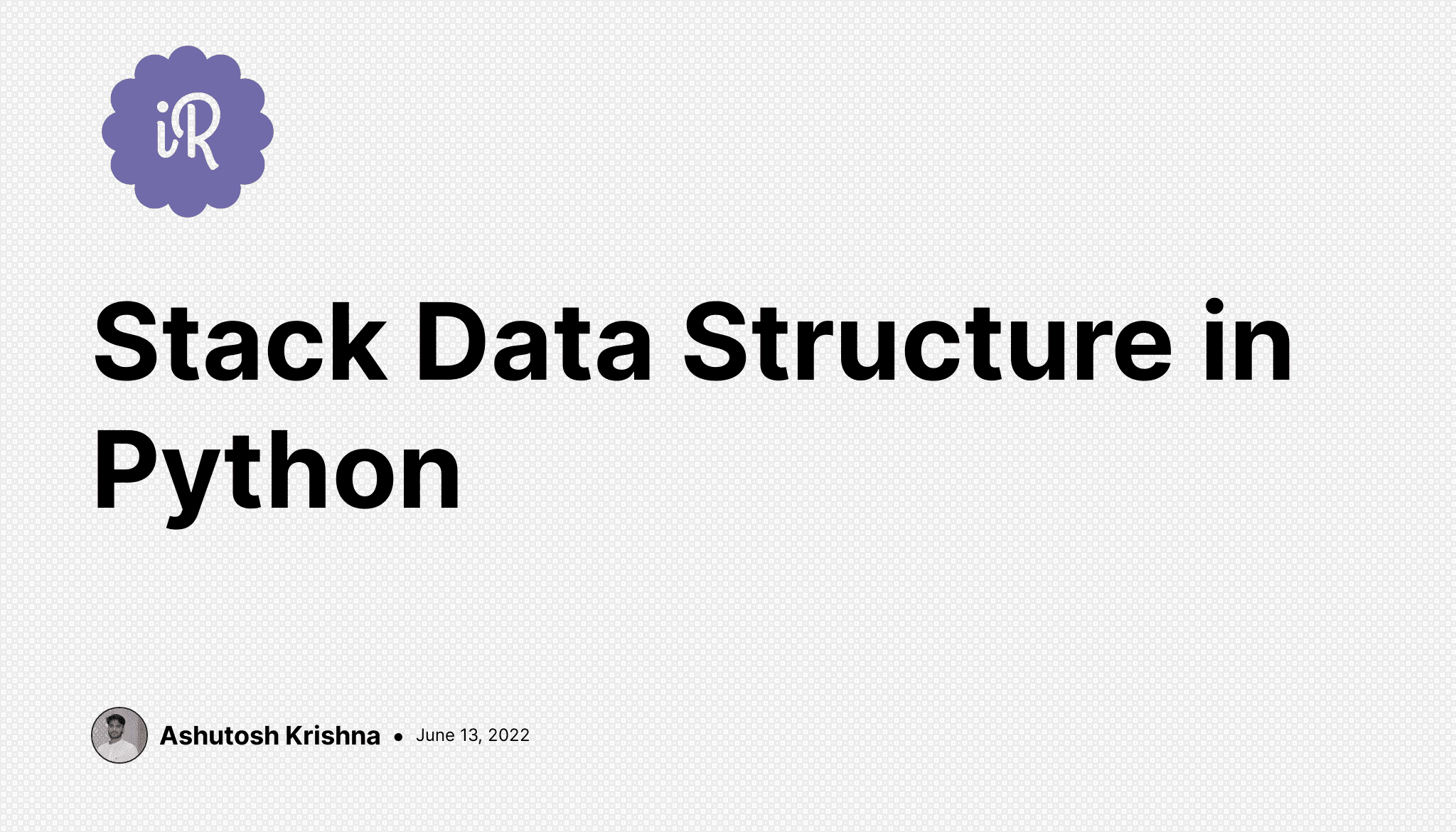# Stack Data Structure in Python

Data Structures DATA STRUCTURES AND ALGORITHMS USING PYTHON SERIESIn this blog, we will discuss Stack data structure. We'll also implement Stack and its different functions using Python. So, let us get started.

# What is Stack?

Stack is one of the linear data structures that follow the Last-In/First-Out (LIFO) principle. You can consider a stack as a pile of books.The book you kept last will be at the top of the pile and will be the first to be taken out. Also, the book that you kept first will be the last one to be taken out. However, you cannot directly take out any random book, let's say the third one. Thus it also follows the LIFO principle.

# Operations on Stack

1. Push: To insert an element in the stack, we use the push operation.
2. Pop: The pop operation removes the topmost element from the stack.
3. Peek: This operation prints the topmost element from the stack. It doesn't remove it.
4. Empty: Checks whether the stack is empty or not.

# Stack Implementation Using List

We can implement a stack using lists in Python. We will create a Stack class using the `list` data structure in Python. If you're not familiar with Python classes, check out this elaborated tutorial on Object-Oriented Programming in Python.

``````class Stack:
pass
``````

Within the Stack class, we will maintain a list called `items`. We can initiate this empty list in the constructor of the Stack class as:

``````class Stack:
def __init__(self):
self.items = []
``````

Now, let us implement the `push()` operation which takes a `data` argument.

``````class Stack:
##...

def push(self, data):
self.items.append(data)
``````

In the `push()` function, we're using the `append()` function to insert the data at the end of the `items` list.

After this, we can implement the `pop()` operation which will remove the topmost element from the stack.

``````class Stack:
##...

def pop(self, data):
return self.items.pop()
``````

Here, we're using the list `pop()` function to remove the last inserted element from the list.

Next, we'll implement the `peek()` operation. As we know this operation returns the topmost element(last inserted element) from the stack, the last element inserted in the list can be accessed using the `items[-1]` since list supports negative indexing.

``````class Stack:
##...

def peek(self):
if not self.is_empty():
return self.items[-1]
``````

The final operation is the `is_empty()` which checks if the stack is empty or not. For this, we can just check if the length of the `items` list is equal to zero or not.

``````class Stack:
##...

def is_empty(self):
return len(self.items) == 0
``````

With this, we have completed the implementation of our Stack class using a list. The Stack class looks like this:

``````class Stack:
def __init__(self):
self.items = []

def push(self, data):
self.items.append(data)

def pop(self):
return self.items.pop()

def peek(self):
if not self.is_empty():
return self.items[-1]

def is_empty(self):
return len(self.items) == 0

def __str__(self):
return str(self.items)
``````

Additionally, we have implemented the `__str__()` function to print the stack.

# Example

To test whether it is working fine or not, we can create an object of this Stack class and perform the various operations on it. So, let us create a separate `test.py` file and try.

``````from stack import Stack

my_stack = Stack()

print(my_stack.is_empty())

my_stack.push(1)
my_stack.push(2)
my_stack.push(3)
print(my_stack)

my_stack.pop()
print(my_stack)

print(my_stack.peek())

popped_element = my_stack.pop()
print(f"Popped Element: {popped_element}")
print(my_stack)
print(my_stack.is_empty())
``````

You can dry run the above operations as well as run the `test.py` file to match your output. The output should be like this:

``````True
[1, 2, 3]
[1, 2]
2
Popped Element: 2

False
``````

# Application of Stack

There are various applications of stack data structure. You have even used some of them.

1. Undo feature
2. Checking if brackets are balanced or not
3. Evaluating expressions

# Conclusion

In this article, we have discussed the Stack data structure and implemented it using a list in Python. There are other ways to implement a Stack as well. We'll discuss them in some other blog. Hope the tutorial was clear. Thanks!